Previous: 2.2.1 Macroscopic Observables Up: 2.2.1 Macroscopic Observables Next: 2.2.1.2 Physical Quantities

### 2.2.1.1 Sets of Moments

The description of the distribution function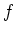by a set of moments eliminates the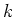-coordinates at the expense of information loss concerning the details of the distribution function, as the common simplification is to investigate only a few moments of the distribution function.

In the parabolic case the energy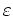is(2.20)

In this special case we can choose the observables to be polynomials in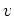.

We set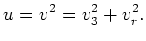(2.21)

and we define the set of velocity observables: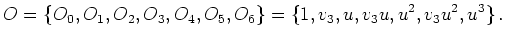(2.22)

The moments derived from these observables are denoted by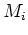. This set of moments naturally corresponds to the use of a shifted'' distribution function (diffusion approximation) as defined in Equation 2.17.

For the non-parabolic case the following set of observables is more appropriate and is used in [GJK+04]: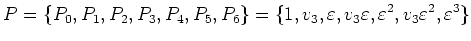(2.23)

We define the energy'' moments as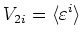(2.24)

and the fluxes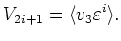(2.25)

In the parabolic band case the energy and the velocity set of moments are equivalent descriptions. In this case the quotient between the velocity moments and the energy moments depends on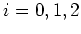. We have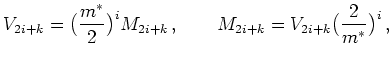(2.26)

where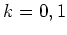.